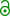## Regulatory acceptance of PowerTOST [Software]

Hi BE-proff,

» In Russia we have two options to prove sample size: either demonstrate calculations with formulas …

See this presentation (slide 8). The procedure is always iterative (since the t-value in the formula to calculate power depends on the degrees of freedom and hence, the sample size itself). The approximation1 by the shifted central t-distribution can be done with a pocket calculator (alternatively: paper-pencil-brain) and a t-table. The better approximation (noncentral t) already needs software, since no tables exist for a range of the noncentrality parameter. The exact method needs numeric integration of two integrals → software.

» … or provide literature reference.

What do you mean by that?

» The 1st option is not for me because I don't have relevant background so I'd prefer the 2nd way.
»
» So, if somebody could share any literature reference in a journal (preferrable) which mentions PowerTOST I'd really appreciate itJones & Kenward2

The power and sample size for a bioequivalence trial can conveniently be calculated using the R package PowerTOST (Labes (2013)).a
The power is calculated using the R package PowerTOST (Labes (2013)) with the “exact” option.b
Using the PowerTOST (Labes (2013)) R library function, the power, for a true ratio of 1, for this estimated CV and a total sample size of 20 is just over 0.04.c

PowerTOST is mentioned in Nadarajah et al.3

In the folder \library\PowerTOST\tests you find R-scripts to validate the various sample size functions against published data. See the man-pages of ct5.1, ct5.2, ct5.3, ct5.4.1, ct9.6.2, ct9.6.4, ct9.6.6, ct9.6.8, ctCW.III, ctSJ.VIII.10, and ctSJ.VIII.20 for the references.

1. Hauschke, D, Steinijans, VW, Diletti E, Burke M. Sample Size Determination for Bioequivalence Assessment Using a Multiplicative Model.
J Pharmacokin Biopharm 1992;20(5);557–61. doi:10.1007/BF01061471.
2. Jones B, Kenward MG. Design and Analysis of Cross-Over Trials.
Boca Raton: CRC Press / Chapman & Hall; 3rd ed. 2015.
1. p.329
2. p.373
3. p.379
3. Nadarajah S, Sergey Bityukov S, Krasnikov N. Confidence distributions: A review.
Stat Meth. 2015;22:23–46. doi:10.1016/j.stamet.2014.07.002.open source.

Dif-tor heh smusma 🖖
Helmut SchützThe quality of responses received is directly proportional to the quality of the question asked. 🚮
Science QuotesIng. Helmut Schütz